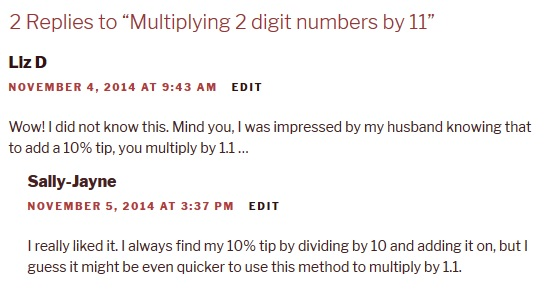Recently we looked at how to square two-digit numbers ending in 5 in your head.  What about the other square numbers?  Well, there’s a quick way to do that in your head as well.

Let’s look at 372.
First of all round the 37 up or down to the nearest 10.
You added 3 to get 40, so you also need to subtract 3 to get 34 (37-3=34)
Multiply these to numbers together 40×34 = 4 x 34 x 10 = 1360 (4×30=120,  4×4=16, 120 +16 = 136, 136×10=1360)
Finally, add on the square of the number you added and subtracted (here it was 3 and 3×3=9 so add 9) 1360+9=1369.
So 372 = 1369

Here’s another example: 622
Round this down to 60, and then because you subtracted 2, you also have to add 2 so you get 60×64 (6 x 64 x 10). 3 x 60 = 360, 6 x 4 = 24, 360 + 24 = 384, 384 x 10 = 3840
Remember to add the square of the number you rounded down by. 22 = 4 so add 4.
3840 + 4 = 3844
So 622 = 3844

If you’ve enjoyed these tricks then you might enjoy the Secrets of Mental Maths course from The Great Courses. It’s fascinating!

## How to square 2 digit numbers ending in 5 (in your head)

This is another trick that my family already knew, but I only learnt it recently so I’m sharing it for anybody out there who doesn’t already know.

Squaring 2 digit numbers is easy if they end in 5.

5×5 = 25, so the last 2 digits of the answer will always be 25.

To find the first digits of the answer, take the first digit of the number you are squaring and multiply it by the next ten up.

Eg 65 x 65

The first digit is 6. The next ten up is 7, so to find the first digits of the answer you multiply 6×7.

6×7 = 42

The last two digits will always be 25 so 652 = 4225

352 = 1225  (3×4 = 12 and the last 2 digits are 25)

852 – 7225 (8×9 = 72 and the last 2 digits are 25)

It’s especially useful for squaring something-and-a-half eg 7.5 x 7.5. 7 x 8 = 56. The last two digits will be 2 and 5, but here you are using ½ not a whole number, so it won’t be 25 but .25 so the answer is 56.25.  3.52 is 12.25 and so on.

If you enjoyed this trick, you might also enjoy this post on how to multiply 2 digit numbers by 11 in your head.

## Multiplying 2 digit numbers by 11

Earlier this year I discovered a trick for multiplying 2 digit numbers by 11.  I taught this to some of the children I tutor to help them work out 11×11 and 11×12 which they had been struggling with but then as it works for all 2 digit numbers they have enjoyed baffling their family and friends with their ability to perform calculations such as 54 x 11 in their head.

Apparently it’s not as big a secret as I had thought (well, everyone in my family already knew how), but I’m going to share it anyway just in case some of you don’t already know how…

First of all you split the digits of the two digit number, and these become the first and last digits of the answer. Eg with 54 x 11, the answer is going to start with 5 and end with 4. To get the number in the middle you just add the two digits together. 5 + 4 = 9, so put 9 in the middle. Your answer is 594.

35 x 11 = 3 [3+5] 5   that is 385
42 x 11 = 4 [4+2] 2   that is 462.

That’s all very well, but what if the two digits add up to more than 9?  That’s easy too. You partition that number, the units become the middle digit and the tens get added to the first digit.

86 x 11 = 8 [8+6] 68  6 → uh oh!  It can’t be 8146. That’s ok – split the 14. The 4 becomes the middle digit, and the ten (1) gets added to the first digit → 8+1=9, so the answer is 946.

Happy calculating!